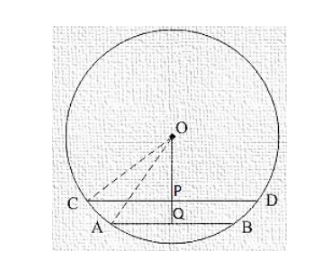# Two chords AB, CD of lengths 5 cm, 11 cm respectively of a circle are parallel.Question:

Two chords AB, CD of lengths 5 cm, 11 cm respectively of a circle are parallel. If the distance between AB and CD is 3 cm, find the radius of the circle.

Solution:

Construction: Draw OP⊥ CD

Chord AB = 5 cm

Chord CD = 11cm

Distance PQ = 3 cm

Let OP = x cm

And OC = OA = r cm

We know that the perpendicular from centre to chord bisects it.

∴ CP = PD = 11/2 cm

And AQ = BQ = 5/2 cm

In ΔOCP, by Pythagoras theorem

$O C^{2}=O P^{2}+C P^{2}$

$\Rightarrow r^{2}=x^{2}+(11 / 2)^{2} \ldots(\mathrm{i})$

In ΔOQA, by Pythagoras theorem

$O A^{2}=O Q^{2}+A Q^{2}$

$\Rightarrow r^{2}=(x+3)^{2}+(5 / 2)^{2} \ldots$ (ii)

Compare equation (i) and (ii)

$\Rightarrow(x+3)^{2}+(5 / 2)^{2}=x^{2}+(11 / 2)^{2}$

$\Rightarrow x^{2}+6 x+9+25 / 4=x^{2}+121 / 4$

$\Rightarrow x^{2}-x^{2}+6 x=121 / 4-25 / 4-9$

⇒ 6x = 15

⇒ x = 15/6 = 5/2